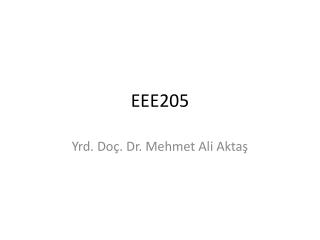DownloadDownload PresentationEEE205

# EEE205

Télécharger la présentation## EEE205

- - - - - - - - - - - - - - - - - - - - - - - - - - - E N D - - - - - - - - - - - - - - - - - - - - - - - - - - -
##### Presentation Transcript

1. EEE205 Yrd. Doç. Dr. Mehmet Ali Aktaş

2. UNITS ASSOCIATED WITH BASICELECTRICAL QUANTITIES • The system of units used in engineering and science isthe (International systemof units), usually abbreviated asSI units.

3. UNITS ASSOCIATED WITH BASICELECTRICAL QUANTITIES • Derived SI units use combinations of basic units andthere are many of them. Two examples are: • Velocity – metres per second (m/s) • Acceleration – metres persecondsquared(m/s2)

4. UNITS ASSOCIATED WITH BASICELECTRICAL QUANTITIES • SI unitsmay bemade larger or smaller by using prefixeswhich denote multiplication or divisionby a particularamount. The six most common multiples, with theirmeaning, arelistedbelow:

5. UNITS ASSOCIATED WITH BASICELECTRICAL QUANTITIES • CHARGE • The unit of charge is the coulomb (C) where onecoulomb is one ampere second (1 coulomb = 6.24×1018 electrons). The coulomb is defined as the quantityof electricity which flows past a given point in an electriccircuit when a current of one ampere is maintainedforonesecond. charge, in coulombsQ=I.t I is the current in amperes, t is the time insec.

6. UNITS ASSOCIATED WITH BASICELECTRICAL QUANTITIES • Problem 1. If a current of 5A flows for 2minutes,find the quantity of electricity transferred. • Quantity of electricity Q = I t coulombs • I = 5 A, t = 2×60 = 120 s • HenceQ= 5×120 = 600C

7. UNITS ASSOCIATED WITH BASICELECTRICAL QUANTITIES • Force • The unit of force is the newton (N) where one newtonis one kilogram metre per second squared. The newtonis defined as the force which, when applied to a mass ofone kilogram, gives it an acceleration of one metre persecond squared. force, in newtonsF=ma • where m is the mass in kilograms and a is the accelerationin metres per second squared.

8. UNITS ASSOCIATED WITH BASICELECTRICAL QUANTITIES • Problem 2. A mass of 5000g is accelerated at2m/s2 by a force. Determine the force needed. • Force = mass×acceleration • = 5kg×2m/s2= 10 kgm/s2 = 10N.

9. UNITS ASSOCIATED WITH BASICELECTRICAL QUANTITIES • Problem 3. Find the force acting verticallydownwards on a mass of 200 g attached to a wire. Mass=200g=0.2 kg and acceleration due to gravity,g=9.81m/s2 (Gravitationalforce, or weight, is mg, where g = 9.81m/s2.) • Force actingdownwards = weight • =mass×acceleration • = 0.2kg×9.81m/s2 • = 1.962N

10. UNITS ASSOCIATED WITH BASICELECTRICAL QUANTITIES • Work The unit of work or energy is the joule (J) where onejoule is one newton metre. The joule is defined as thework done or energy transferred when a force of onenewton is exerted through a distance of onemetre in thedirection of theforce. work done on a body, in joules, W=Fs where F is the force in newtons and s is the distance inmetres moved by the body in the direction of the force.Energy is the capacity for doing work.

11. UNITS ASSOCIATED WITH BASICELECTRICAL QUANTITIES • Power The unit of power is thewatt (W) where onewattis onejoule per second. Power is defined as the rate of doingworkortransferringenergy. power, in watts, P= W / t where W is the work done or energy transferred, injoules, and t is the time, in seconds. energy, in joules, W=Pt

12. UNITS ASSOCIATED WITH BASICELECTRICAL QUANTITIES • Problem 4. A portablemachinerequires a forceof 200N to move it. How much work is done if themachine is moved 20m and what average power isutilized if the movement takes 25s? Work done = force×distance = 200N×20m =4 000Nm or 4 kJ Power = work done /time taken =4000J / 25s =160J/s= 160W